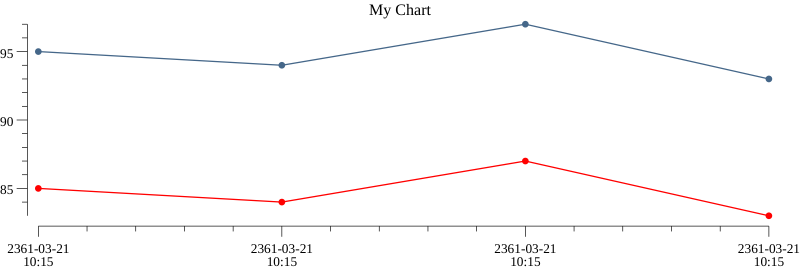# chart

Core API

This module available in Core API

Module `chart` allows to create an image with a chart. After you can upload an image to remote storage and attach to an alert.

Usage:

script.lua
``````local chart = require('chart')
``````

## Methods

### render

`render('<CHART_TITLE>', <CHART_OPTIONS>) binaryImageData, error`

Render image and return it as binary data

If an error occurred, it will be returned as second value

## Chart Options

A structure `<CHART_OPTIONS>` describe data for build a chart

``````{
series = { <CHART_SERIES>, <CHART_SERIES>, ... }
}
``````

Series is lines on a chart

### Series

``````{
color = '<COLOR>',
line_color = '<COLOR>',
point_color = '<COLOR>',
data = { <POINT_VALUE>, <POINT_VALUE>, ... }
}
``````

`color`, `line_color`, `point_color` allows define a common color, a line color or a point color

If defined only `color` - it will be applying to lines and points Default color is black

`data` contains data for every points

One point describe as:

``````{
timestamp = <FLOAT>
value = <FLOAT>
}
``````

### Color

`color` (`color_line`, `color_point`) may have:

One of these values - blue - red - black - green - yellow

String `#XXXXXX` for define a color in format RGB or `#XXXXXXXX` for define a color in format RGBA

An example:

``````color = 'blue'
color = '#00FF00'
color = '#ABCD12FA'
``````

## Examples

Manually define a chart data:

script.lua
``````local chartOptions = {
['series'] = {
{
['color'] = '#456789',
["data"] = {
{ ['timestamp'] = 12345646554, ['value'] = 95 },
{ ['timestamp'] = 12345646555, ['value'] = 94 },
{ ['timestamp'] = 12345646556, ['value'] = 97 },
{ ['timestamp'] = 12345646557, ['value'] = 93 }
}
},
{
['color'] = 'red',
["data"] = {
{ ['timestamp'] = 12345646554, ['value'] = 85 },
{ ['timestamp'] = 12345646555, ['value'] = 84 },
{ ['timestamp'] = 12345646556, ['value'] = 87 },
{ ['timestamp'] = 12345646557, ['value'] = 83 }
}
}
}
}

local img, err = chart.render('My Chart', chartOptions)

-- 'img' will be contains an image binary data
``````

Result imageObtain a data from Prometheus (Usage metrics from NodeExporter)

script.lua
``````local rangeOptions = {
['start'] = r - 86400,
['end'] = r,
['step'] = '3600'
}

res1 = prom.range('rate(sum(node_cpu_seconds_total{mode!="idle",node="32"}[5m])) / rate(sum(node_cpu_seconds_total{node="32"}[5m]))', rangeOptions)
res2 = prom.range('rate(sum(node_cpu_seconds_total{mode!="idle",node="43"}[5m])) / rate(sum(node_cpu_seconds_total{node="43"}[5m]))', rangeOptions)

local chartOptions = {
['series'] = {
{
['color'] = '#456789',
["data"] = res1.values,
},
{
['color'] = 'red',
['line_color'] = 'blue',
["data"] = res2.values,
}
}
}

local img, err = chart.render('CPU', chartOptions)

-- 'img' will be contains an image binary data
``````

Result image## Send image

You can store received image to the upload storage and then use image URL.

For telegram channel you can send raw image data without upload to the storage